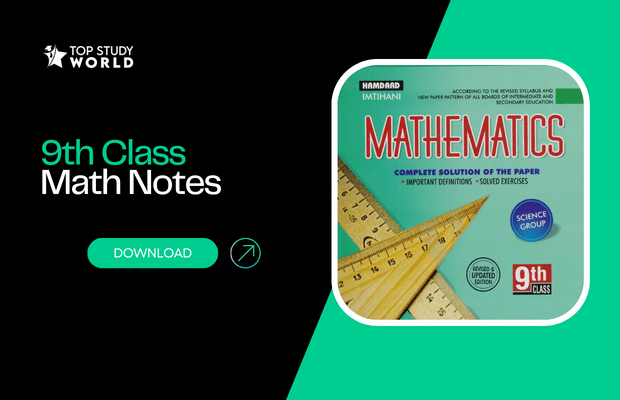Are you a student of class 9th and looking for the 9th Class Maths notes with solutions for the complete book? The notes dedicated to the FBISE students are here. Here is your Class 9 Maths solved Notes with Example Solutions For Federal Board in PDF free.

All Subjects 9th Class Notes in One Place

Most Pakistani students consider mathematics a difficult subject because they don’t take this subject with the other subjects. Rather having a firm belief in “parrot fashioning (Ratta’lization).” So, such a subject that fits differently in your study must have a special administration also.

The best way is to practice as much as possible. Revise theorems every day because they are more likely to be forgotten.

Revising every day makes sense. When you memorize a topic the next day, about 20 to 30% fades away from your mind, especially in maths, where a lot of practice is required.

## CHAPTER 1: MATRICES AND DETERMINANTS

Topics that chapter 1 includes are:

• Matrix:
• Types of Matrices
• Addition and Subtraction of Matrices
• Multiplication of Matrices
• Multiplicative Inverse of a Matrix
• Solution of Simultaneous Linear Equations

• Size: 7 MB | Pages: 53

## CHAPTER 2: REAL AND COMPLEX NUMBERS

Topics that chapter 2 includes are:

• Real Numbers
• Properties of Real Numbers
• Laws of Exponents/Indices
• Complex Numbers
• Basic Operations on Complex Numbers

• Size: 2.3 MB | Pages: 11

## CHAPTER 3: LOGARITHMS

Topics that chapter 3 includes are:

• Scientific Notation
• Logarithm
• Common Logarithm and Natural Logarithm
• Laws of Logarithm
• Application of Laws of Logarithm in Calculations

• Size: 2.9 MB | Pages: 19

## CHAPTER 4: ALGEBRAIC EXPRESSIONS AND ALGEBRAIC

Topics that chapter 4 includes are:

• Algebraic Expressions
• Algebraic Formula
• Surds and their Application
• Rationalization of Surds

• Size: 3.6 MB | Pages: 27

## CHAPTER 5: FACTORIZATION

Topics that chapter 5 includes are:

• Factorization
• Remainder Theorem and Factor Theorem
• Factorization of a Cubic Polynomial

• Size: 3.7 MB | Pages: 23

## CHAPTER 6: ALGEBRAIC MANIPULATION

Topics that chapter 6 includes are:

• Highest Common Factor & Least Common
• Basic Operations on Algebraic Fractions
• Square Root of Algebraic Expression

• Size: 3.5 MB | Pages: 23

## CHAPTER 7: LINEAR EQUATIONS AND INEQUALITIES

Topics that chapter 7 includes are:

• Linear Equations
• Equations Involving Absolute Value
• Linear Inequalities
• Solving Linear Inequalities

• Size: 3.2 MB | Pages: 23

## CHAPTER 8: LINEAR GRAPHS & THEIR APPLICATION

Topics that chapter 8 includes are:

• Cartesian Plane and Linear Graphs
• Conversion Graphs
• Graphically Solving 2 Variable Linear

• Size: 3.1 MB | Pages: 17

## CHAPTER 9: INTRODUCTION TO COORDINATE GEOMETRY

Topics that chapter 9 includes are:

• Distance Formula
• Collinear Points
• Mid-Point Formula

• Size: 2 MB | Pages: 17

## CHAPTER 10: CONGRUENT TRIANGLES

• Size: 2.2 MB | Pages: 16

## CHAPTER 11: PARALLELOGRAMS AND TRIANGLES

• Size: 2.6 MB | Pages: 17

## CHAPTER 12: LINE BISECTORS AND ANGLE BISECTORS

• Size: 1.5 MB | Pages: 10

## CHAPTER 13: SIDES AND ANGLES OF A TRIANGLE

• Size: 1.8 MB | Pages: 11

## CHAPTER 14: PYTHAGORAS THEOREM

• Size: 2.1 MB | Pages: 15

## CHAPTER 15: PARALLELOGRAMS AND TRIANGLES

• Size: 1.4 MB | Pages: 9